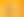Operating with Decimals

# Stages1. Decimal Number Sense

EXPLORE

Suggested Learning Intentions

• To understand decimal place value to the thousandths
• To understand the relative size of decimals

Sample Success Criteria

• I can describe decimal place value to thousandths
• I can explain and justify my solutions using a variety of manipulatives such as place value blocks, counters or decimats
• I can use my knowledge of place value to compare, order and round decimals
EXPLORE

EXPLORE

Suggested Learning Intentions

• To solve decimal problems using rounding and estimation
• To use my understanding of place value to solve decimal problems

Sample Success Criteria

• I can round appropriately based on the context
• I can explain the reasonableness of my estimations when working with decimals
• I can rename decimals to assist with computation
• I can model my solution using manipulatives such as place value blocks or decimats
• I can apply my knowledge of addition and subtraction strategies when working with decimals
EXPLORE

3. Multiplying and Dividing by Powers of 10

EXPLORE

Suggested Learning Intentions

• To understand that scientific notation can be used to describe infinitely large and infinitely small numbers
• To learn how to multiply and divide decimals using knowledge of place value in a base-10 system

Sample Success Criteria

• I can multiply and divide decimals by powers of 10 using written and mental strategies
• I can describe different kinds of numbers using scientific notations
• I can justify my thinking and solutions using manipulatives
EXPLORE

4. Multiplying and Dividing Decimals

EXPLORE

Suggested Learning Intentions

• To recognise the appropriate operation when solving decimal problems in context
• To use my number sense when multiplying and dividing decimal problems

Sample Success Criteria

• I can select the operation and use effective strategies when solving decimal problems
• I can explain my thinking using pictures, diagrams and number sentences when solving decimal problems
• I can explain and justify my solutions using manipulatives or other representations of the mathematics
EXPLORE